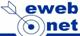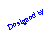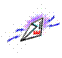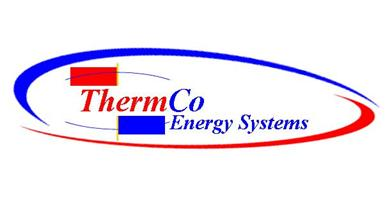Wet Bulb Calculation
 Here is an example:   Suppose the temperature is 42 degrees Fahrenheit with a dew-point of 15 degrees Fahrenheit. The dew-point depression is 42-15 = 27. Now divide 27 by 3 = 9. Now subtract 9 from the original temperature of 42. 42 - 9 = 33.   For temperatures between 30 and 60 degrees F, the 1/3 rule works quite well. For warmer temperatures than 60, the cooling is between about 1/3 and 1/2 the dew-point depression.     If the temperature was 42 with a dew-point of 15 and it started raining, the temperature and dew-point would wet-bulb out to a chilly 33 degrees Fahrenheit. As dew-point depression or temperature increase, the evaporation potential increases. This technique does not give the exact wet bulb temperature but it does give a pretty close approximation. Warmer air will cool at a greater rate than colder air since more water vapor can evaporate into warm air. Evaporation is a cooling process that absorbs latent heat, therefore the more evaporation the more cooling.A quick technique that many forecasters use to determine the wet-bulb temperature is called the "1/3 rule". The technique is to first find the dew-point depression (temperature minus dew-point). Then take this number and divide by 3. Subtract this number from the temperature. You now have an approximation for the wet-bulb temperature.